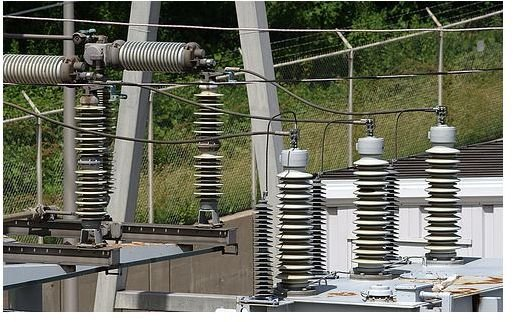# Power Factor Correction Transformers & Power Factor Calculations

All electrical equipment works with a degree of efficiency which may be poor or excellent. The efficiency of electrical equipment like transformers and induction motors is indicated by the term power factor. In the technical world, the power factor is defined as ratio of the true power of the equipment and the apparent power.

If you want to understand the calculation of power factor and its correction, you need to understand a few terms, which we'll discuss here.

• KW is the amount of power by which the transformer does useful work. It is also called active power, real power, or actual power.
• KVAR is used to denote reactive power. This is the power that the transformer needs to develop magnetized flux.
• KVA denotes the apparent power. It is the vector sum of reactive power and actual power.

As mentioned above, the power factor is equal to the working power and apparent power.

Power Factor= KW / KVA

Therefore, we can say that the value of power factor is always less than one and depends upon the value of KVA. The more the value of KVA, the less will be the value of power factor.

## Power Factor Correction Using Capacitor

To determine the value of KVA we use the right angle rule. It is square root of the sum of KW and KVAR.KVA= Sqrt (KW2 + KVAR2)

To increase the power factor, we need to reduce the amount of required KVA. To do so, we have to shorten the line of KVAR, and this work is done by introducing a capacitor. The capacitor subtracts the extra KVAR and improves the power factor.

These capacitors are available on the market based on how much KVAR value you need to reduce to get the desired power factor.

Let we take a look how the calculation of the correction is done.

First of all we need to measure the amount of apparent power or KVA required for the transformer to work. After getting this value, you need to determine what the power factor of the transformer is and how much power factor you want to get.

Suppose, your transformer requires 560 KVA to operate and it has the power factor 0.55. You want to increase this power factor to 0.95.

You need to use the formula given below to determine the value of KVAR.

KVAR = KVA*(1-pf2)

Now, follow the three steps given below to determine how much KVAR value you need to reduce.

• Calculate the value of KVAR using value of the present power factor.

KVAR1 = 560*(1-0.552)

KVAR1 = 467.

• Calculate the value of KVAR using desired value of power factor.

KVAR2 = 560*(1-0.952)

KVAR2 = 175

• Now, subtract KVAR1 by KVAR2. You will get 292 KVAR.

You can use this value to purchase the exact capacitor so as you would get the desired value of power factor.

You can also use the power factor table to determine the required reduction in KVAR. Follow the steps given below to use this table.

• Determine the original power factor of the transformer and also the desired power factor.
• Get the value in the table where the row of original power factor and column of desired power factor meet.
• Determine the value of KW. If you have KVA value, divide the KVA by the original power factor to get KW.
• Now multiply KW with the value you have gotten in the table. This is the KVAR that is required to reduce.

## Important Points to Increase the Power Factor

• The power factor may reduce if the transformer works under a lighter load. Moreover, the efficiency of the transformer reduces if it is used below or above its load range, but lighter load affects it more. You have to operate the transformer under the load range for which it is made. It will increase the power factor and service life of the transformer.
• You have to operate the transformer at rated voltage. If you don’t follow this instruction, the service life of the transformer will definitely be reduced and the power factor, too. Using the transformer at rated voltage would increase the power factor with time.

## Summary

This is how the transformer power factor calculation and correction is done to increase efficiency. Use of a capacitor is a very effective way of correcting or improving the power factor of transformers and other electrical equipment. This method is cheap; however, it should be done only by a professional electrician. You should always operate the transformer at the rated voltage and load range to avoid any reduction in service life and power factor.

## References

Reference- Power Factor- The Basics

Reference-Power Factor Calculation

Image- Transformer- Flickr, by- Beige Alert